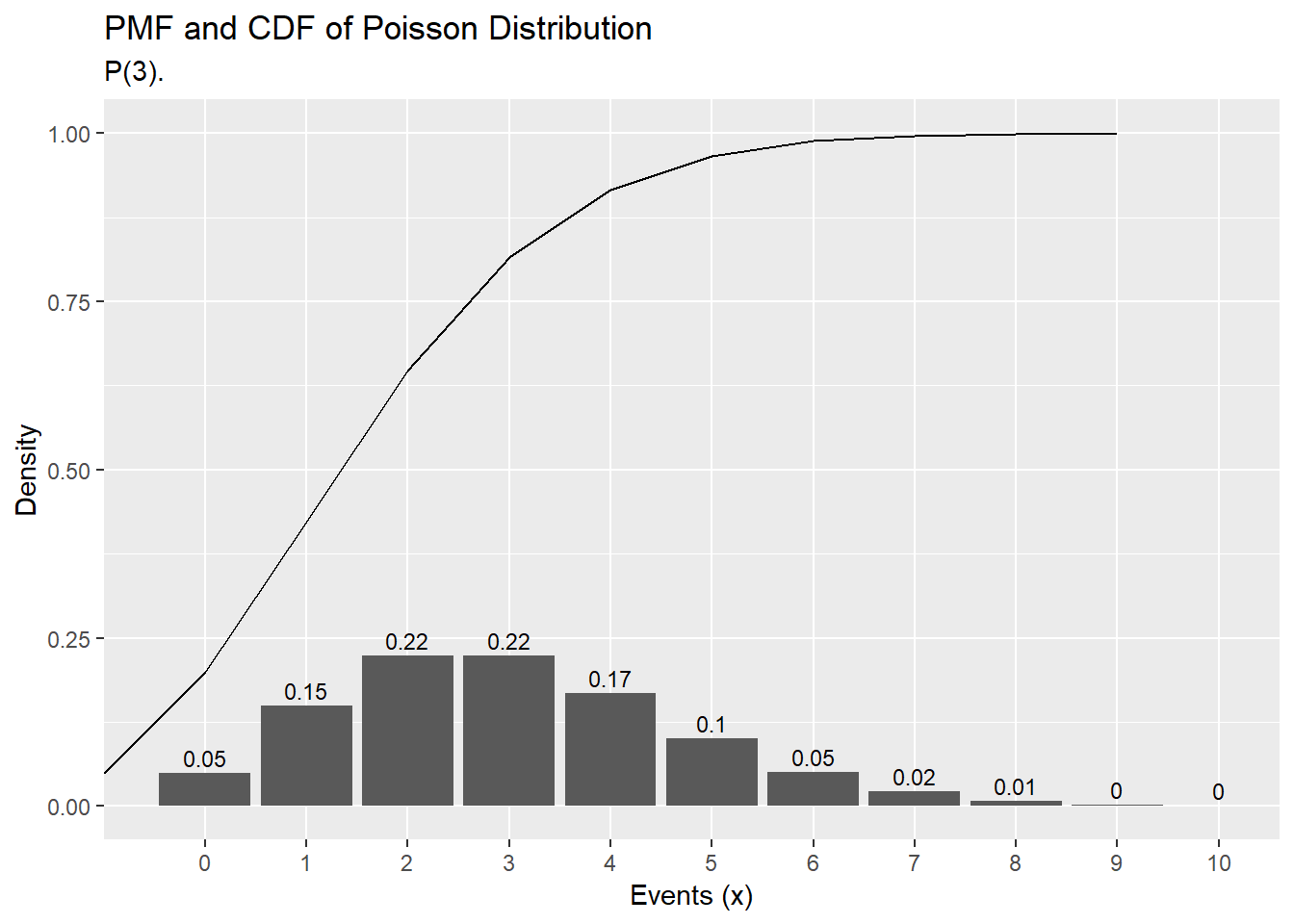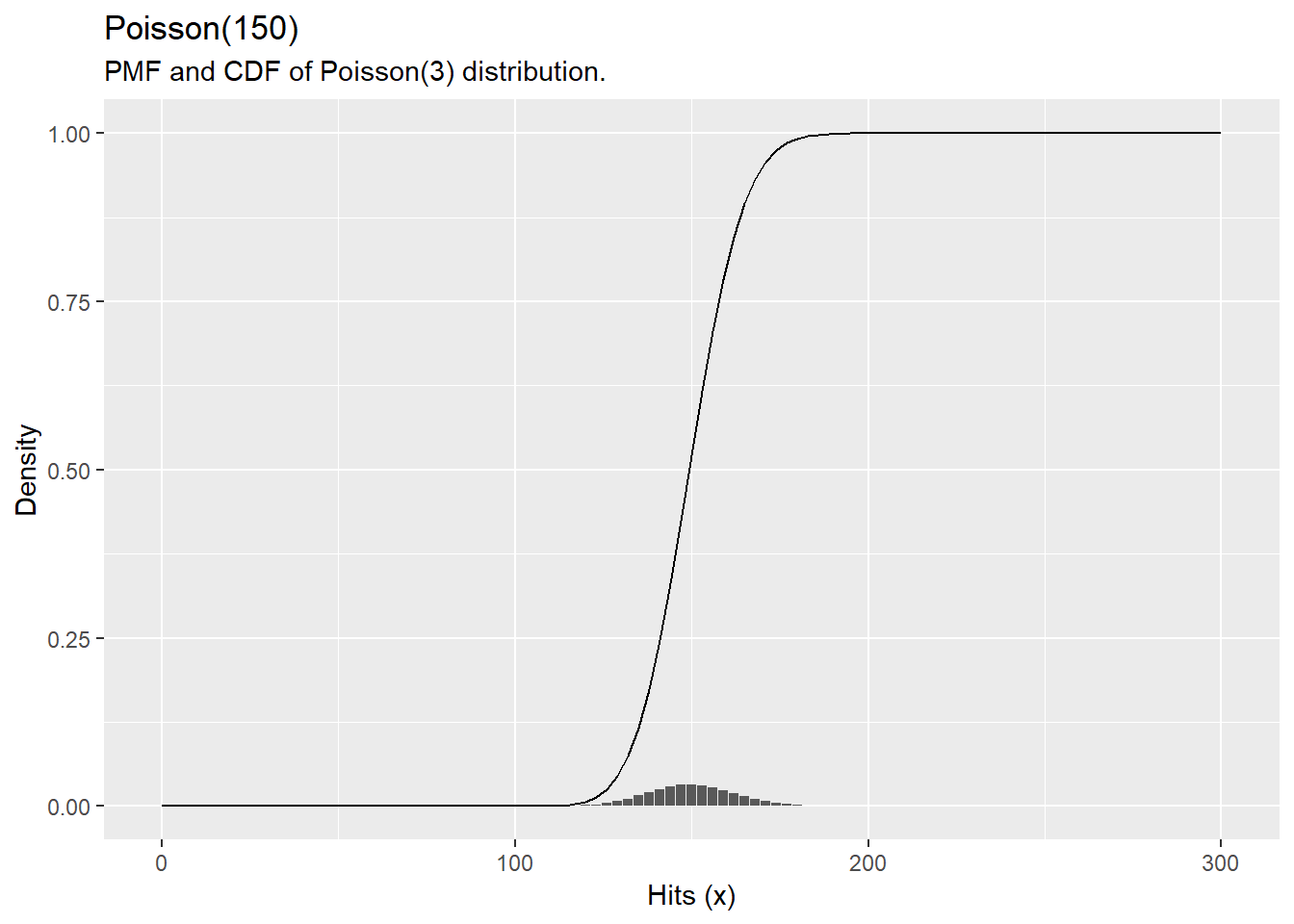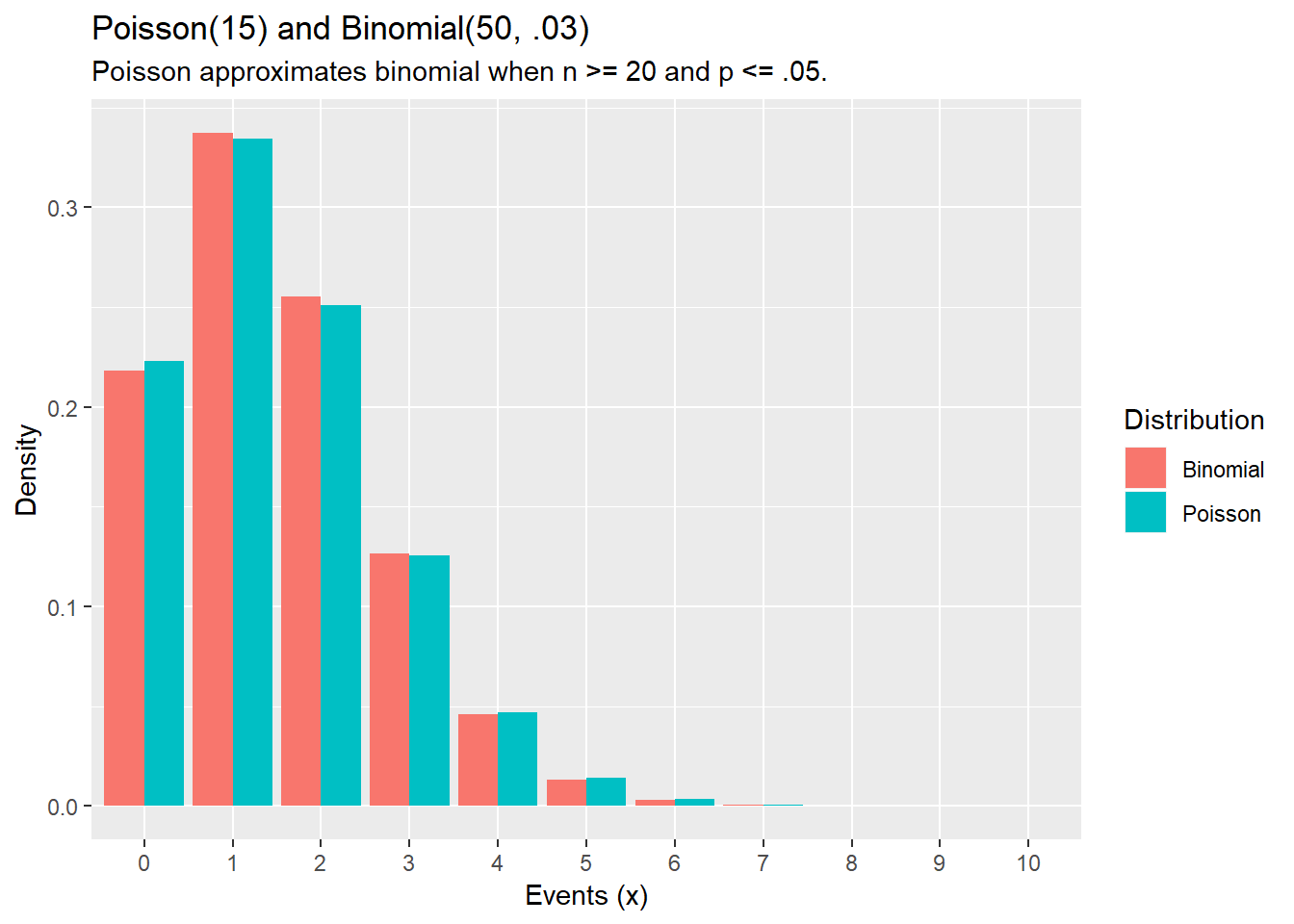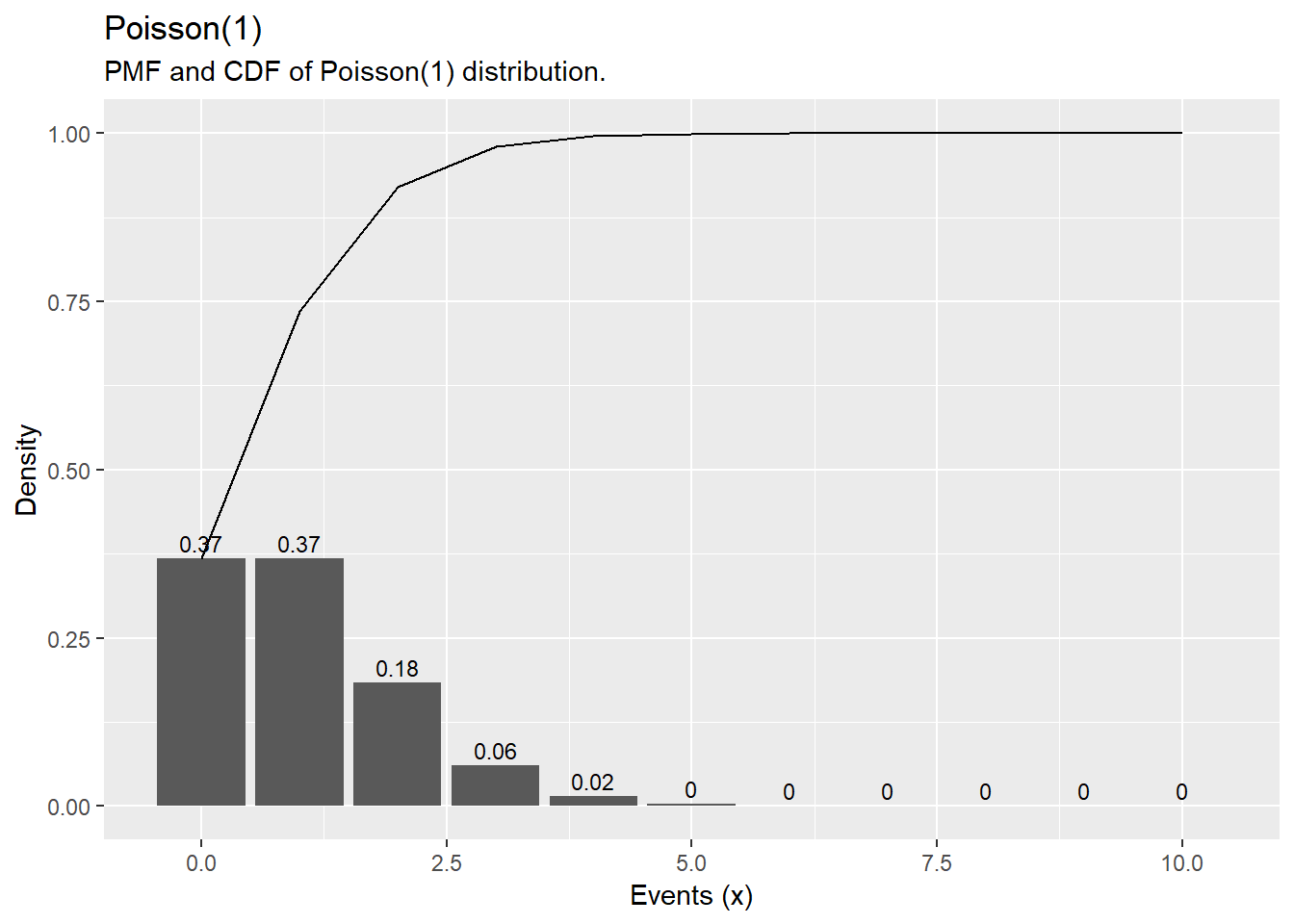# R Functions dpois, ppois, and rpois

Random varaible $$X$$ is distributed $$X \sim P(\lambda)$$ with mean $$\mu=\lambda$$ and variance $$\sigma^2 = \lambda$$ if $$X = x$$ is the number of successes in $$n$$ (many) trials when the probability of success $$\lambda / n$$ is small. The probability of $$X = k$$ successes is $$Pr(X = k) = (e^{-\lambda} \lambda^k)/k!$$.

R function dpois(x, lambda) is the probability of x successes in a period when the expected number of events is lambda. R function ppois(q, lambda, lower.tail) is the cumulative probability (lower.tail = TRUE for left tail, lower.tail = FALSE for right tail) of less than or equal to q successes. R function rpois(n, lambda) returns n random numbers from the Poisson distribution x ~ P(lambda). R function qpois(p, lambda, lower.tail returns the value (quantile) at the specified cumulative probability (percentile) p.

#### Example

What is the probability of making 2 to 4 sales in a week if the average sales rate is 3 per week?

# Using cumulative probability
ppois(q = 4, lambda = 3, lower.tail = TRUE) -
ppois(q = 1, lambda = 3, lower.tail = TRUE)
##  0.616115
# Using exact probability
dpois(x = 2, lambda = 3) +
dpois(x = 3, lambda = 3) +
dpois(x = 4, lambda = 4)
##  0.6434504
# expected number of sales = lambda = 3

# variance = lambda = 3

library(ggplot2)
library(dplyr)
options(scipen = 999, digits = 2) # sig digits

events <- 0:10
density <- dpois(x = events, lambda = 3)
prob <- ppois(q = events, lambda = 3, lower.tail = TRUE)
df <- data.frame(events, density, prob)
ggplot(df, aes(x = factor(events), y = density)) +
geom_col() +
geom_text(
aes(label = round(density,2), y = density + 0.01),
position = position_dodge(0.9),
size = 3,
vjust = 0
) +
labs(title = "PMF and CDF of Poisson Distribution",
subtitle = "P(3).",
x = "Events (x)",
y = "Density") +
geom_line(data = df, aes(x = events, y = prob))#### Example

Suppose a baseball player has a p=.300 batting average. What is the probability of X<=150 hits in n=500 at bats? X=150? X>150?

# probability of x <= 150
ppois(q = 150, lambda = .300 * 500, lower.tail = TRUE)
##  0.52
# probability of x = 150
dpois(x = 150, lambda = .300 * 500)
##  0.033
# probability of x > 150
ppois(q = 150, lambda = .300 * 500, lower.tail = FALSE) 
##  0.48
library(ggplot2)
library(dplyr)
options(scipen = 999, digits = 2) # sig digits

hits <- 0:100 * 3
density <- dpois(x = hits, lambda = .300 * 500)
prob <- ppois(q = hits, lambda = .300 * 500, lower.tail = TRUE)
df <- data.frame(hits, density, prob)
ggplot(df, aes(x = hits, y = density)) +
geom_col() +
labs(title = "Poisson(150)",
subtitle = "PMF and CDF of Poisson(3) distribution.",
x = "Hits (x)",
y = "Density") +
geom_line(data = df, aes(x = hits, y = prob))The Poisson distribution approximates the binomial distribution with $$\lambda=np$$ if $$n>=20$$ and $$p<=0.05$$.

#### Example

What is the distribution of successes from a sample of n = 50 when the probability of success is p = .03?

library(ggplot2)
library(dplyr)
library(tidyr)
## Warning: package 'tidyr' was built under R version 3.4.4
options(scipen = 999, digits = 2) # sig digits

n = 0:10
df <- data.frame(events = 0:10,
Poisson = dpois(x = n, lambda = .03 * 50),
Binomial = dbinom(x = n, size = 50, p = .03))
df_tidy <- gather(df, key = "Distribution", value = "density", -c(events))
ggplot(df_tidy, aes(x = factor(events), y = density, fill = Distribution)) +
geom_col(position = "dodge") +
labs(title = "Poisson(15) and Binomial(50, .03)",
subtitle = "Poisson approximates binomial when n >= 20 and p <= .05.",
x = "Events (x)",
y = "Density")#### Example

Suppose the probability that a drug produces a certain side effect is p = = 0.1% and n = 1,000 patients in a clinical trial receive the drug. What is the probability 0 people experience the side effect?

# The expected value is np
1000 * .001
##  1
# The probability of measuring 0 when the expected value is 1
dpois(x = 0, lambda = 1000 * .001) 
##  0.37
library(ggplot2)
library(dplyr)
options(scipen = 999, digits = 2) # sig digits

x <- 0:10
density <- dpois(x = x, lambda = 1000 * .001)
prob <- ppois(q = x, lambda = 1000 * .001, lower.tail = TRUE)
df <- data.frame(x, density, prob)
ggplot(df, aes(x = x, y = density)) +
geom_col() +
geom_text(
aes(label = round(density,2), y = density + 0.01),
position = position_dodge(0.9),
size = 3,
vjust = 0
) +
labs(title = "Poisson(1)",
subtitle = "PMF and CDF of Poisson(1) distribution.",
x = "Events (x)",
y = "Density") +
geom_line(data = df, aes(x = x, y = prob))